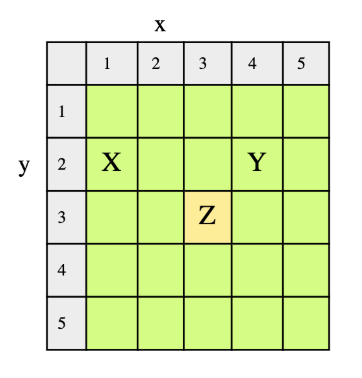# Closest Meeting Point

## Problem Statement

Given N people on an MxM grid, find the point that requires the least total distance covered by all people to meet at that point.

Consider a 5x5 grid with 3 people; one at X(1,2), Y(4,2) and Z(3,3).We need to find the meeting point(x,y) for these people where the total distance covered by all three is the minimum. They can travel in all directions: horizontally, vertically, and diagonally. The minimum distance point, in this case, is (3,3).

## Hint

• Distance between two points
• Centroid of a two-dimensional region

## Try it yourself

point shortest_distance_travelled(int m,    vector<point> points) {  point pt(1, 1);  //TODO: Write - Your - Code  return pt;}

## Solution

class point {private:  int x;  int y;public:  point(int x, int y) {    this->x = x;    this->y = y;  }  int getX() const {    return x;  }  void setX(int x) {    this->x = x;  }  int getY() const {    return y;  }  void setY(int y) {    this->y = y;  }  double calculate_distance(point p) {    double distance;    distance = sqrt(        (p.x - this->x) * (p.x - this->x)            + (p.y - this->y) * (p.y - this->y));    return distance;  }  double calculate_sum_of_distances(vector<point> points) {    double distance_sum;    distance_sum = 0;    for (int i = 0; i < points.size(); i++) {      distance_sum += this->calculate_distance(points[i]);    }    return distance_sum;  }};point shortest_distance_travelled_2(int m,    vector<point> points) {  point min_pt(0, 0);  double x = 0;  double y = 0;  // calculate the centroid  point centroid(0, 0);  for (int i = 0; i < points.size(); i++) {    x += points[i].getX();    y += points[i].getY();  }  centroid.setX((int) round(x / points.size()));  centroid.setX((int) round(y / points.size()));  // initialize the min_pt to centroid  min_pt.setX(centroid.getX());  min_pt.setY(centroid.getY());  double min_distance = min_pt.calculate_sum_of_distances(      points);  // checking points surrounding the potential centroid  for (int i = min_pt.getX() - 1; i < min_pt.getX() + 2;      i++) {    for (int j = min_pt.getY() - 1; j < min_pt.getY() + 2;        j++) {      if (i < 1 || j > m) {        continue;      }      point pt(i, j);      double distance = pt.calculate_sum_of_distances(          points);      if (distance < min_distance) {        min_distance = distance;        min_pt.setX(pt.getX());        min_pt.setY(pt.getY());      }    }  }  return min_pt;}void test_grid1() {  cout << "Testing grid 1...\n\n";  int m = 5;	//size of the grid  vector<point> points;  points.push_back(point(1, 2));  points.push_back(point(3, 3));  points.push_back(point(4, 2));  cout << "Solution 2:\n";  point pt = shortest_distance_travelled_2(m, points);  cout << "Shortest Distance Point = p(" << pt.getX()      << ", " << pt.getY() << ")" << endl;}void test_grid2() {  cout << "Testing grid 2...\n\n";  int m = 10;	//size of the grid  vector<point> points;  points.push_back(point(1, 1));  points.push_back(point(3, 5));  points.push_back(point(6, 2));  points.push_back(point(7, 7));  points.push_back(point(8, 4));  cout << "Solution 2:\n";  point pt = shortest_distance_travelled_2(m, points);  cout << "Shortest Distance Point = p(" << pt.getX()      << ", " << pt.getY() << ")" << endl;}void test_grid3() {  cout << "Testing grid 3...\n\n";  int m = 8;	//size of the grid  vector<point> points;  points.push_back(point(4, 3));  points.push_back(point(5, 5));  points.push_back(point(2, 7));  cout << "Solution 2:\n";  point pt = shortest_distance_travelled_2(m, points);  cout << "Shortest Distance Point = p(" << pt.getX()      << ", " << pt.getY() << ")" << endl;}int main() {  test_grid1();  test_grid2();  test_grid3();  cout << "\nCompleted!" << endl;  return 0;}

## Solution Explanation

### Runtime Complexity

Linear, O(n).

Here ‘n’ is the number of people on the grid.

### Memory Complexity

Linear, O(n).

Here ‘n’ is the number of people on the grid.

### Solution Breakdown

This solution uses a ‘centroid’ to find the minimum distance travelled point.

The centroid of a two-dimensional region is the arithmetic mean or average position of all the points. We can calculate the centroid of all the points with people on the grid and that will be the minimum distance travelled point. It can be calculated as follows:

centroid = $(\frac{x1+x2+x3+...+xn}{n},$ $\frac{y1+y2+y3+...+yn}{n})$

It is the average of x-coordinates and y-coordinates.

In most cases, the centroid is the correct answer but, in some cases, the centroid gives the point closest to the correct point. So, to handle this case, we’ll first find the centroid and then check its surrounding 8 points for possible minimum distance travelled point. If any of those points has a distance less than the distance at the centroid, then that point will be the minimum distance travelled point.

The reason why the centroid sometimes gives an incorrect point is that we basically take the average of the all the points and then round off the value, irrespective of the minimum distance covered by all points. This will definitely return a point lying in the middle of all the points, which in most cases will be correct. But in some cases, the closest point may lie at any of the input points, which we will never be able to get using the Centroid formula. So, we double-check the neighbors of the “closest point” to handle this scenario.

Let’s understand this with the help of an example. Consider the example 5x5 grid with 3 people at (1,2), (3,3) and (4,2).

x = $\frac{1+3+4}{3}$ = $\frac{8}{3}$ = 3

y = $\frac{2+3+2}{3}$ = $\frac{7}{3}$ = 2

centroid = (3,2)

Here, we’re using the round values giving x=3. After checking all surrounding points of the centroid, it appears that at point (3,3), the sum of distances covered by all people is less than the distance at the centroid. So eventually, (3,3) becomes the minimum distance travelled point.

Practice problems like this and many more by checking out our Grokking the Coding Interview: Patterns for Coding Questions course!

Learn in-demand tech skills in half the time